# Poincaré return map

(diff) ← Older revision | Latest revision (diff) | Newer revision → (diff)

successor mapping, of a smooth or at least continuous flow (continuous-time dynamical system)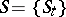and a hypersurfacetransversal to it

A mappingthat assigns to a point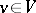that point of the intersection ofand the positive semi-trajectory of the flow starting atthat comes first in time (it is defined for thosefor which such an intersection exists). (The hypersurfaceis called a section, an intersecting surface or a transversal.) If the dimension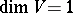(so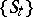is a flow in the plane or on a two-dimensional surface,is called a contactless arc) and ifis parametrized by a numerical parameter, then the shift of points ofunder the Poincaré return map is described by some numerical function in one variable (ifcorresponds to the parameter value, thencorresponds to the parameter value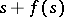), which is called the successor function. This mapping was used for the first time by H. Poincaré (see ), that is why it is called the Poincaré return map.

If all semi-trajectories intersect, then the Poincaré return map (in this case defined on the whole of) determines the behaviour of all trajectories of the flow to a considerable extent. However, such "global" sections are far from being common (in particular, a Hamiltonian system on a manifold of constant energy which does not pass through critical points of the Hamiltonian, i.e. through equilibrium positions (cf. Equilibrium position), does not have closed — as manifolds — global sections, see , Chapt. 8, Sect. 4.7).

For a non-autonomous system with a periodic right-hand side,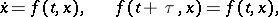(*)

there exists an analogue of the Poincaré return map: to the pointcorresponds the point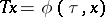, where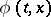is the solution of (*) with initial value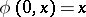. This "map of the shift by a period" can be considered even formally as a Poincaré return map if (*) is considered as an autonomous system in "cylindrical" phase space. The mapis defined everywhere if the solutions of (*) are defined for all.

More often one has to deal with a "local" section — it is cut only by a part of the trajectories and often only part of the trajectories intersecting it return again to. As an example one can consider a small smooth "surface element" of codimension 1 intersecting transversally some periodic trajectory. In this case the Poincaré return map is defined near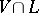and characterizes the behaviour of the trajectories near.

In the theory of foliations one can also introduce a Poincaré return map (see ), which is a generalization of the above example (and includes the Poincaré return map for ordinary differential equations in a complex domain).

How to Cite This Entry:
Poincaré return map. Encyclopedia of Mathematics. URL: http://encyclopediaofmath.org/index.php?title=Poincar%C3%A9_return_map&oldid=16330
This article was adapted from an original article by D.V. Anosov (originator), which appeared in Encyclopedia of Mathematics - ISBN 1402006098. See original article